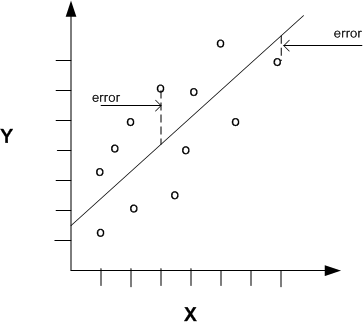# Simple Regression in R

In this post, we will look at how to perform a simple regression using R. We will use a built-in dataset in R called ‘mtcars.’ There are several variables in this dataset but we will only use ‘wt’ which stands for the weight of the cars and ‘mpg’ which stands for miles per gallon.Developing the Model

We want to know the association or relationship between the weight of a car and miles per gallon. We want to see how much of the variance of ‘mpg’ is explained by ‘wt’. Below is the code for this.

`> Carmodel <- lm(mpg ~ wt, data = mtcars)`

Here is what we did

1. We created the variable ‘Carmodel’ to store are information
2. Inside this variable, we used the ‘lm’ function to tell R to make a linear model.
3. Inside the function, we put ‘mpg ~ wt’ the ‘~’ sign means ’tilda’ in English and is used to indicate that ‘mpg’ is a function of ‘wt’. This section is the actual notation for the regression model.
4. After the comma, we see ‘data = mtcars’ this is telling R to use the ‘mtcar’ dataset.

Seeing the Results

Once you pressed enter you probably noticed nothing happens. The model ‘Carmodel’ was created but the results have not been displayed. Below is various information you can extract from your model.

The ‘summary’ function is useful for pulling most of the critical information. Below is the code for the output.

```> summary(Carmodel)

Call:
lm(formula = mpg ~ wt, data = mtcars)

Residuals:
Min      1Q  Median      3Q     Max
-4.5432 -2.3647 -0.1252  1.4096  6.8727

Coefficients:
Estimate Std. Error t value Pr(>|t|)
(Intercept)  37.2851     1.8776  19.858  < 2e-16 ***
wt           -5.3445     0.5591  -9.559 1.29e-10 ***
---
Signif. codes:  0 ‘***’ 0.001 ‘**’ 0.01 ‘*’ 0.05 ‘.’ 0.1 ‘ ’ 1

Residual standard error: 3.046 on 30 degrees of freedom
Multiple R-squared:  0.7528,	Adjusted R-squared:  0.7446
F-statistic: 91.38 on 1 and 30 DF,  p-value: 1.294e-10```

We are not going to explain the details of the output (please see simple regression). The results indicate a model that explains 75% of the variance or has an r-square of 0.75. The model is statistically significant and the equation of the model is -5.45x + 37.28 = y

Plotting the Model

We can also plot the model using the code below

```> coef_Carmodel<-coef(Carmodel)
> plot(mpg ~ wt, data = mtcars)
> abline(a = coef_Carmodel, b = coef_Carmodel)```

Here is what we did

1. We made the variable ‘coef_Carmodel’ and stored the coefficients (intercept and slope) of the ‘Carmodel’ using the ‘coef’ function. We will need this information soon.
2. Next, we plot the ‘mtcars’ dataset using ‘mpg’ and ‘wt’.
3. To add the regression line we use the ‘abline’ function. To add the intercept and slope we use a = the intercept from our ‘coef_Carmodel’ variable which is subset  from this variable. For slope, we follow the same process but use a . This will add the line and your graph should look like the following.From the visual, you can see that as weight increases there is a decrease in miles per gallon.

Conclusion

R is capable of much more complex models than the simple regression used here. However, understanding the coding for simple modeling can help in preparing you for much more complex forms of statistical modeling.